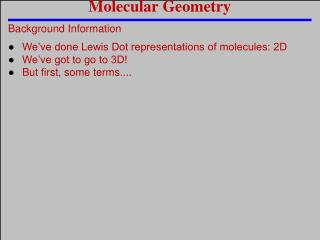# Molecular Geometry - PowerPoint PPT PresentationDownload PresentationMolecular Geometry

Molecular GeometryDownload Presentation## Molecular Geometry

- - - - - - - - - - - - - - - - - - - - - - - - - - - E N D - - - - - - - - - - - - - - - - - - - - - - - - - - -
##### Presentation Transcript

1. Molecular Geometry Background Information • We’ve done Lewis Dot representations of molecules: 2D • We’ve got to go to 3D! • But first, some terms....

2. Molecular Geometry Some Terms: • Electron Domains: The spaces electrons occupy around the nucleus of an atom • Bonding domains: shared pairs • Any bond type = 1 bonding domain • Non-bonding domains: lone pairs

3. Molecular Geometry Some Terms - Electron Domains O H O C H C H H

4. Molecular Geometry • VSEPR Theory Valence Shell Electron Pair Repulsion Theory - Electron domains repel each other • They push as far from each other as possible • VSEPR theory + electron domains = 3D structures

5. Molecular Geometry One Last Thing to Keep in Mind • Not all electron domains are the same size: -Bonded electron domains are long and skinny -Due to the pull of the two nuclei -Non-bonding electron domains are short and fat -Due to the pull of one nuclei - + + - +

6. Molecular Geometry Central Atoms with 4 Electron Domains • When four objects surround a central point, what angles are created if these objects are as far apart as possible? 109.5º angles Tetrahedral

7. Molecular Geometry 4 Electron Domains: Tetrahedral domain geometry Molecule LDMS Bonding Angle Shape Name Non-Bonding H H C H 4 0 109.5º CH4 Tetrahedral H Trigonal Pyramid N H H <109.5º 1 3 NH3 H <109.5º H O H Bent 2 2 H2O HF Straight F H 1 3 Undefined Let’s see it! http://phet.colorado.edu/en/simulation/build-a-molecule

8. Molecular Geometry Central Atoms with 4 Electron Domains • All of these molecules have tetrahedral domain geometries -They all have 4 electron domains • Their molecular geometries depend on the number of bonding and non-bonding domains

9. Molecular Geometry Central Atoms with 3 Electron Domains • When three objects surround a central point, what angles are created if these objects are as far apart as possible? 120.0º angles Trigonal Planar

10. Molecular Geometry 3 Electron Domains: Trigonal Planar domain geometry Molecule LDMS Bonding Non-Bonding Angle Shape Name H C C H 3 0 120.0º Trigonal Planar C2H4 H H 2 1 O N O NO2-1 Bent < 120.0º • Both of these molecules have trigonal planar domain geometries -They both have 3 electron domains Let’s see it! http://phet.colorado.edu/en/simulation/build-a-molecule

11. Molecular Geometry Central Atoms with 3 Electron Domains • Their molecular geometries depend on the number of bonding and non-bonding domains • Notice that we left off the example of 1 bonding pair - Anytime there is only one bonding pair, the angle is undefined and the name is straight, regardless of the number of electron domains

12. Molecular Geometry Central Atoms with 2 Electron Domains ●When two objects surround a central point, what angles are created if these objects are as far apart as possible? 180.0º angles Linear

13. Molecular Geometry 2 Electron Domains: Linear domain geometry Molecule LDMS Bonding Non-Bonding Angle Shape Name 2 0 180.0º CO2 O O C Linear • Any molecule with 2 electron domains will have a linear domain geometry • Notice, again, that we didn’t include an example with 1 bonding domain Let’s see it! http://phet.colorado.edu/en/simulation/build-a-molecule

14. Molecular Geometry Let’s Practice • Draw the LDMS for the following molecules. Then… • Identify the domain and molecular geometries • Determine the bond angle of the central atom PBr3 O2 Trigonal pyramid Straight Undefined DG = MG = Angle = O O Br Br P HCN Br H C N Tetrahedral Trigonal pyramid 107.0º DG = MG = Angle = Linear Linear 180.0º DG = MG = Angle =